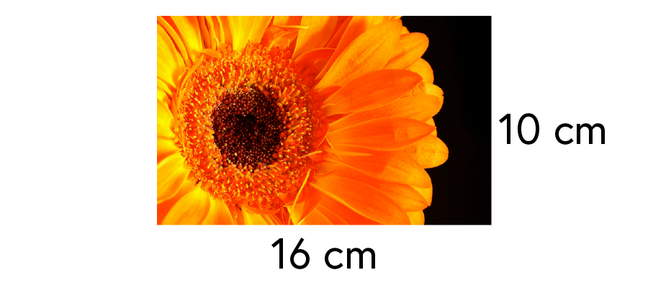## Want to keep learning?

This content is taken from the National STEM Learning Centre's online course, Maths Subject Knowledge: Proportional Reasoning. Join the course to learn more.
1.2

## National STEM Learning Centre

Skip to 0 minutes and 1 second [Paula] So in our example here we have got two photographs, and one is an enlargement of another. They are both in proportion to each other, and we know that because we can compare their height and the base. [Michael] The original height was 10 centimetres and the base was 16 centimetres. So for all of these questions that involve proportional reasoning, what we are going to try and do is draw a diagram to help us understand where the numbers are coming from, and hopefully make the relationship between the numbers a little bit more apparent. So what I’m going to do is take the information given for the original picture, the height was 10 centimetres and the base was 16 centimetres.

Skip to 0 minutes and 52 seconds Then in our final bit here, we had the height being 30 and the base being 48. So I’m going to write those numbers in the boxes below. So this is going to be 30, and that is going to be 48. [Paula] So what do we use these boxes for then? [Michael] Well the ones in our examples, in the grey boxes, they are going to be what we multiply by to get from one box to another box. Or if we are working backwards, it could be something that we divide by. So in this example, because the height was 10, it’s now 30. To get from 10 to 30, we are going to multiply by 3.

Skip to 1 minute and 28 seconds So our linear scale factor is three, it got three times taller. Now because we kept the same proportion, we do exactly the same to the base, so it was 16 and we are now going to multiply by three, and thankfully, that does give us our 48. These things have been enlarged in proportion. [Paula] So by using the clear diagram, we can see the information from our question, and it’s a structure we can use for linear scale factor, area scale factor and ratio proportion. [Michael] Yes, I think the important thing is being sure that you are consistent in your approach.

Skip to 2 minutes and 3 seconds That you can actually see that a lot of these ratio and proportion questions, they are all the same type of thing. They all have the same underlying structure. So by setting it out in a consistent way, it actually makes the questions a lot more easy.

# Introduction to proportional reasoning

Proportional reasoning is to do with scaling up and scaling down quantities. In week 1 we explore techniques which will help you solve a variety of different problems.

In this video we introduce proportional reasoning by looking at enlarging a photograph. When a photograph is enlarged, the height and the base of the photograph are multiplied by the same number. If the height and base are multiplied by different numbers the photograph will be stretched and the picture will become distorted.The height and base can be multiplied by any number.

Think about what will happen if the height and base are multiplied by:
a) 2
b) 1
c) 0.5
d) 1.5